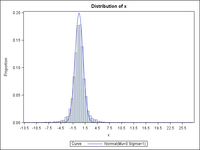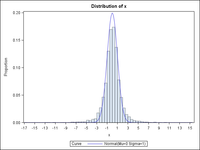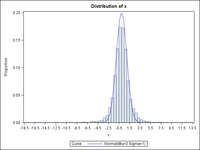## ENDPOINTS in PROC UNIVARIATE Doesn't work

I am drawing multiple histograms using PROC UNIVARIATE as follows. Drawing the histograms, I impose ENDPOINTS to limit the domain and better compare the resulting histograms. Here is the working example.

``````data temp;
do s=1 to 10;
do i=1 to 5000;
x=rand("t",3);
output;
end;
end;
run;
ods graphics on;
axis1 order=(0 to 0.25 by 0.05) minor=none;
proc univariate;
var x;
by s;
histogram/normal(mu=0,sigma=1)
vaxis=axis1
vscale=proportion
endpoints=-5 to 5 by 0.5;
run;
ods graphics off;
quit;``````

Sadly, SAS ignores ENDPOINTS when there are outliers. The code above spits out the following unstable images with respective domains instead.Is WHERE -5<x<5 the only way here? Can I rather force ENDPOINTS to work and detour WHERE? Thank you.

1 ACCEPTED SOLUTION

Accepted Solutions

## Re: ENDPOINTS in PROC UNIVARIATE Doesn't work

If the main concern is the histogram fitting in your desired range:

```proc univariate data=temp noprint;
where -5 le x le 5;
var x;
by s;
histogram/normal(mu=0,sigma=1 noprint)
vaxis=axis1
vscale=proportion
endpoints=-5 to 5 by 0.5;
run;```

If you want the tabular summary to reflect the full data then do not use the histogram in one call.

The above example does not print the tables associated with the proc or the histogram. Remove the NOPRINT if you want the tables.

Be aware that the tables will be filtered by the WHERE statement.

Or use Proc SGPLOT or SGPANEL where there are more controls available

7 REPLIES 7

## Re: ENDPOINTS in PROC UNIVARIATE Doesn't work

What does the log say?

For what you want, you may need SGPLOT instead.

@Junyong wrote:

I am drawing multiple histograms using PROC UNIVARIATE as follows. Drawing the histograms, I impose ENDPOINTS to limit the domain and better compare the resulting histograms. Here is the working example.

``````data temp;
do s=1 to 10;
do i=1 to 5000;
x=rand("t",3);
output;
end;
end;
run;
ods graphics on;
axis1 order=(0 to 0.25 by 0.05) minor=none;
proc univariate;
var x;
by s;
histogram/normal(mu=0,sigma=1)
vaxis=axis1
vscale=proportion
endpoints=-5 to 5 by 0.5;
run;
ods graphics off;
quit;``````

Sadly, SAS ignores ENDPOINTS when there are outliers. The code above spits out the following unstable images with respective domains instead.Is WHERE -5<x<5 the only way here? Can I rather force ENDPOINTS to work and detour WHERE? Thank you.

## Re: ENDPOINTS in PROC UNIVARIATE Doesn't work

If the main concern is the histogram fitting in your desired range:

```proc univariate data=temp noprint;
where -5 le x le 5;
var x;
by s;
histogram/normal(mu=0,sigma=1 noprint)
vaxis=axis1
vscale=proportion
endpoints=-5 to 5 by 0.5;
run;```

If you want the tabular summary to reflect the full data then do not use the histogram in one call.

The above example does not print the tables associated with the proc or the histogram. Remove the NOPRINT if you want the tables.

Be aware that the tables will be filtered by the WHERE statement.

Or use Proc SGPLOT or SGPANEL where there are more controls available

## Re: ENDPOINTS in PROC UNIVARIATE Doesn't work

I think this is the only way. As you mentioned, I may need (1) the sample statistics from the full data and (2) the histograms from the partial data—so NOPRINT suppresses many unwanted numbers in (2). Thanks, but in (1), can I suppress unnecessary histograms? This is the example.

``````resetline;
dm"log;clear;output;clear;graph;end;odsresult;clear;";
option nodate nonumber ls=128 ps=max;
proc datasets lib=work kill nolist;
run;
data _01;
do i=1 to 5000;
x=rand("t",3);
output;
end;
run;
ods select none;
ods results=off;
ods output GoodnessOfFit=_02;
proc univariate data=_01;
var x;
histogram/normal(mu=0,sigma=1);
run;
ods results=on;
ods select all;
proc univariate data=_01 noprint;
var x;
where -5<x<5;
histogram/normal(mu=0,sigma=1,noprint) endpoints=-5 to 5 by 0.25;
run;
quit;``````

While the second UNIVARIATE just produces the histogram I need, the first UNIVARIATE produces both the full sample statistics and the ugly histogram. For the second one, I need to include HISTOGRAM/NORMAL(MU=0,SIGMA=1) to do Kolmogorov–Smirnov, Anderson–Darling, etc. with N(μ=0,σ²=1)—it seems NORMAL in the UNIVARIATE statement just selects the parameters automatically. Is there any similar way such as NOPRINT that suppresses not the tables but the histograms? Thanks.

## Re: ENDPOINTS in PROC UNIVARIATE Doesn't work

@Junyong wrote:

I think this is the only way. As you mentioned, I may need (1) the sample statistics from the full data and (2) the histograms from the partial data—so NOPRINT suppresses many unwanted numbers in (2). Thanks, but in (1), can I suppress unnecessary histograms? This is the example.

``````resetline;
dm"log;clear;output;clear;graph;end;odsresult;clear;";
option nodate nonumber ls=128 ps=max;
proc datasets lib=work kill nolist;
run;
data _01;
do i=1 to 5000;
x=rand("t",3);
output;
end;
run;
ods select none;
ods results=off;
ods output GoodnessOfFit=_02;
proc univariate data=_01;
var x;
histogram/normal(mu=0,sigma=1); <=Delete this line if you don't want a histogram.
run;
ods results=on;
ods select all;
proc univariate data=_01 noprint;
var x;
where -5<x<5;
histogram/normal(mu=0,sigma=1,noprint) endpoints=-5 to 5 by 0.25;
run;
quit;``````

While the second UNIVARIATE just produces the histogram I need, the first UNIVARIATE produces both the full sample statistics and the ugly histogram. For the second one, I need to include HISTOGRAM/NORMAL(MU=0,SIGMA=1) to do Kolmogorov–Smirnov, Anderson–Darling, etc. with N(μ=0,σ²=1)—it seems NORMAL in the UNIVARIATE statement just selects the parameters automatically. Is there any similar way such as NOPRINT that suppresses not the tables but the histograms? Thanks.

Highlighting apparently doesn't work in "running man" code boxes so here:

```proc univariate data=_01;
var x;
histogram/normal(mu=0,sigma=1); <=Delete this line if you don't want a histogram.
run;
```

## Re: ENDPOINTS in PROC UNIVARIATE Doesn't work

I do need the line for the normality tests such as Kolmogorov–Smirnov. I just need to suppress those ugly histograms (byproducts) from the first UNIVARIATE as the second UNIVARIATE creates what I need.

## Re: ENDPOINTS in PROC UNIVARIATE Doesn't work

Use ODS SELECT <outputName> to control the output.

## Re: ENDPOINTS in PROC UNIVARIATE Doesn't work

It's not clear to me how you want to handle the observations outside of [-5, 5].  If you want to omit them, then, yes, use the WHERE clause.

The documentation for the ENDPOINTS= option says "

The range of endpoints must cover the range of the data. For example, if you specify

```endpoints=2 to 10 by 2
```

then all of the observations must fall in the intervals [2,4) [4,6) [6,8) [8,10].

"

If the data do not fall within the range of the endpoints, the endpoints list is extended until there is a bin for all observations.

Discussion stats
• 7 replies
• 1912 views
• 0 likes
• 4 in conversation